SLIDE RULES:
Space #84 "The Orrery" / Star Center Antique Mall / Snohomish, WA
Email us: starmall@myantiquemall.com / Call us:  360 568-2131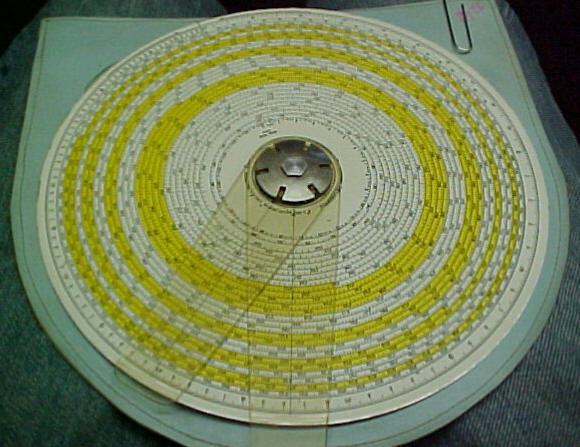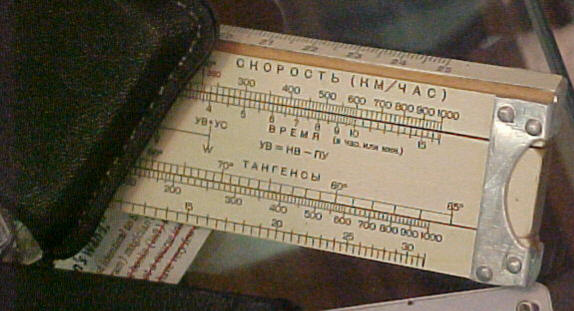Circular Slide Rule SOLD                                                  Russian Slide Rule \$77.50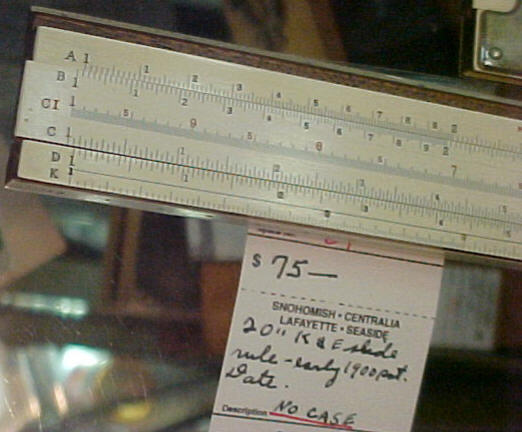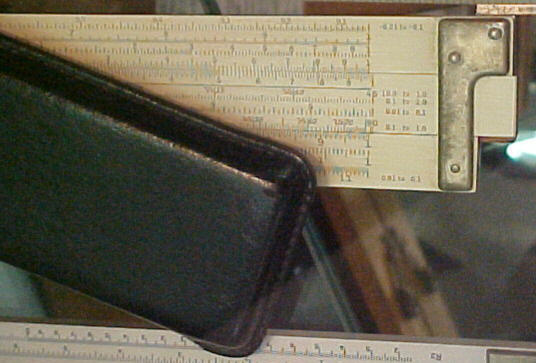K&E (Keuffel + Esser Co) slide rule \$75.00            K+E Slide rule SOLD

History of the Slide Rule:
Many thanks to http://www.hpmuseum.org/sliderul.htm for this great article.

In 1614, John Napier discovered the logarithm which made it possible to perform multiplications and divisions by addition and subtraction. (ie: a*b = 10^(log(a)+log(b)) and a/b = 10^(log(a)-log(b)).)

This was a great time saver but there was still quite a lot of work required. The mathematician had to look up two logs, add them together and then look for the number whose log was the sum. Edmund Gunter soon reduced the effort by drawing a number line in which the positions of numbers were proportional to their logs.

The scale started at one because the log of one is zero. Two numbers could be multiplied by measuring the distance from the beginning of the scale to one factor with a pair of dividers, then moving them to start at the other factor and reading the number at the combined distance.

Picture of a 2 foot Gunter scale (~110K) The yellow spots are brass inserts to provide wear resistance at commonly used points.

Closeup on the Gunter scale (~72K)

Soon afterwards, William Oughtred simplified things further by taking two Gunter's lines and sliding them relative to each other thus eliminating the dividers.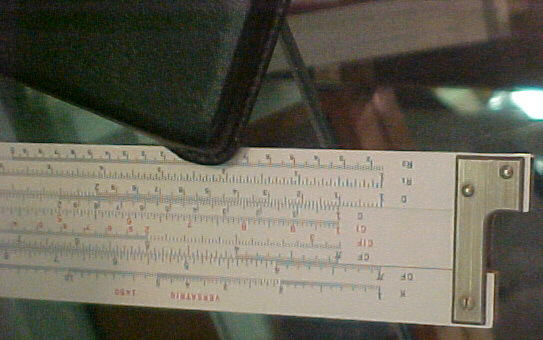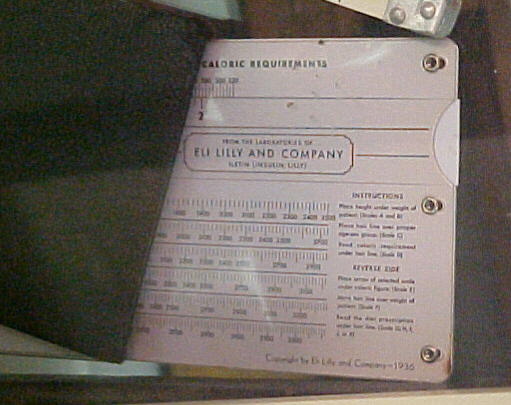Post Slide Rule \$50.00                                                                   Eli and Lilly + Co Slide Rule \$27.50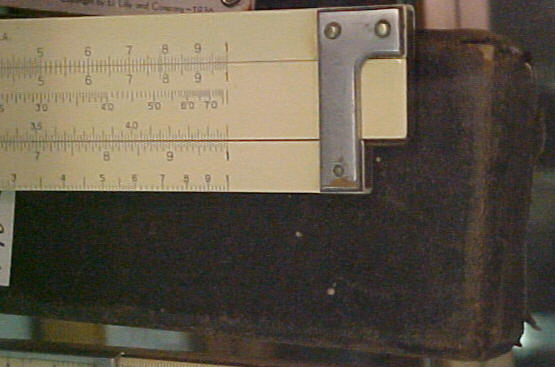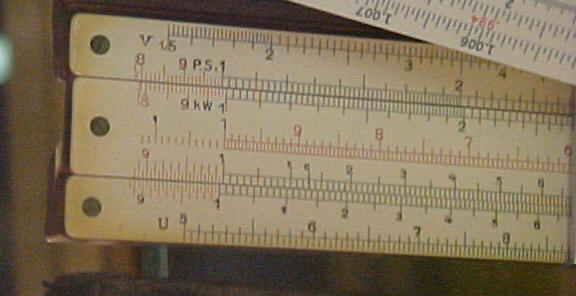Eugene Dietzgen + Co Slide Rule \$40.00             Albert Nestler Slide Rule \$90.00

In the years that followed, other people refined Oughtred's design into a sliding bar held in place between two other bars. Circular slide rules and cylindrical/spiral slide rules also appeared quickly. The cursor appeared on the earliest circular models but appeared much later on straight versions. By the late 17th century, the slide rule was a common instrument with many variations. It remained the tool of choice for many for the next three hundred years.

While great aids, slide rules were not particularly intuitive for beginners. A 1960 Pickett manual said:

"When people have difficulty in learning to use a slide rule, usually it is not because the instrument is difficult to use. The reason is likely to be that they don't understand the mathematics on which the instrument is based, or the formulas they are trying to evaluate. Some slide rule manuals contain relatively exhaustive explanations of the theory underlying the operations. In this manual it is assumed that the theory of exponents, of logarithms, of trigonometry, and of the slide rule is known to the reader, or will be recalled or studied by reference to formal textbooks on these subjects."

A 1948 Stanley manual expressed a somewhat different opinion:

"The principles of logarithmic calculators are too well known to those likely to be interested for it to be necessary to enlarge upon the subject here, especially as it is absolutely unnecessary to have any knowledge of the subject to use the calculator"
...
"Anyone can calculate with the Fuller after a brief study of the following instructions without any mathematical knowledge whatever."

Another interesting quote from the same Pickett manual:

"A computer who must make many difficult calculations usually has a slide rule close at hand."

In 1960, "computer" was still understood to be a person who computed. By contrast, a recent dictionary begins the only definition of "computer" with "An electronic machine..."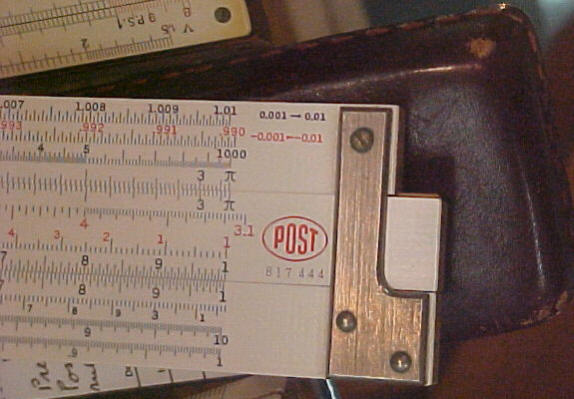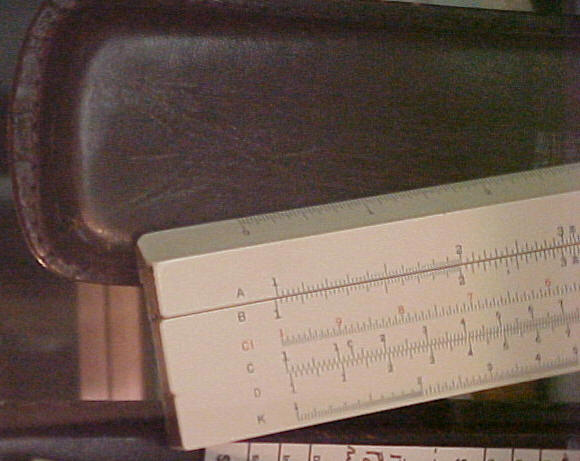Post Slide Rule \$75.00                                                           Sun Hemmi Slide Rule \$28.50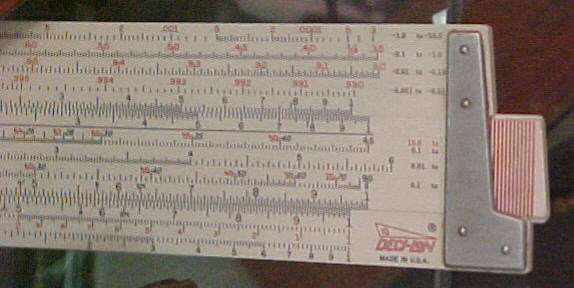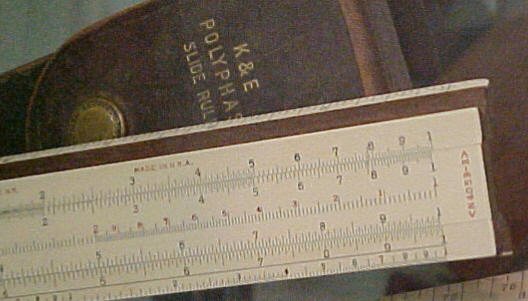K+E Decilon Slide Rule SOLD                                           K+E Slide Rule \$35.00

Some Slide Rule Terms

Mannheim
A standard single-face rule with scales to solve problems in multiplication, division, squares, square roots, reciprocals, trigonometry and logarithms.
Polyphase
Like a Mannheim but added a scale for cubes and cube roots and an inverted C scale (CI) to make certain problems easier to solve. (Some manufacturers used Mannheim and Polyphase interchangeably.)
Phillips
The single sided rule similar to a Polyphase but with and inverted A scale (typically labeled R) instead of an inverted C scale (CI).
Duplex
A double-faced rule. Typically added three folded scales (CF, CIF, DF) to those of the Polyphase rule to make many problems easier to solve.
Trig
A rule with scales for solving trigonometry problems (S, ST, T).
Decitrig
A rule with the trigonometric scales (S, ST, T) marked in degrees and tenths of a degree.
Dual Base
A rule with that read both common and natural logs.
Log Log
A rule with scales for raising numbers to powers. (Scales usually started with LL)
Vector
A rule with hyperbolic functions.
Combinations
The above terms were often combined on more complex rules like Polyphase Duplex Decitrig. (In this case the double-sided duplex overrode the single sided assumption of Polyphase.)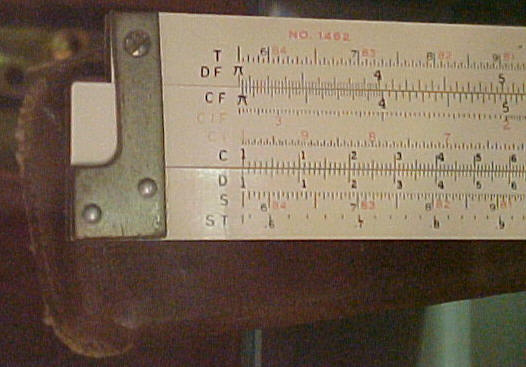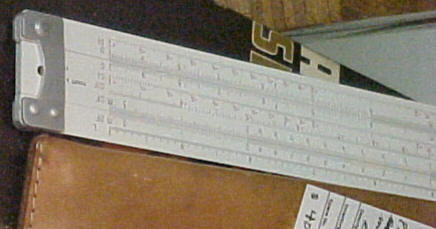Post Slide Rule \$29.95                                           Pickett Slide Rule \$40.00 Click the link above to shop more than 10,000 antiques and view 85+ articles on antiques. Looking for a specific vintage item? Ask us!         360 568-2131 / starmall@myantiquemall.com